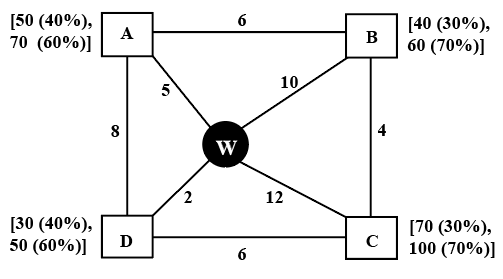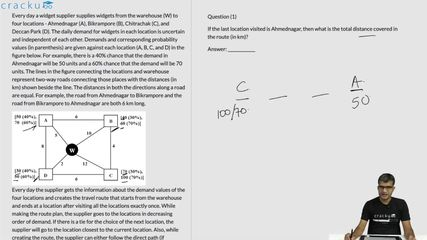### CAT 2022 Question Paper (Slot 2) Question 33

Instructions

Every day a widget supplier supplies widgets from the warehouse (W) to four locations - Ahmednagar (A), Bikrampore (B), Chitrachak (C), and Deccan Park (D). The daily demand for widgets in each location is uncertain and independent of each other. Demands and corresponding probability values (in parenthesis) are given against each location (A, B, C, and D) in the figure below. For example, there is a 40% chance that the demand in Ahmednagar will be 50 units and a 60% chance that the demand will be 70 units. The lines in the figure connecting the locations and warehouse represent two-way roads connecting those places with the distances (in km) shown beside the line. The distances in both the directions along a road are equal. For example, the road from Ahmednagar to Bikrampore and the road from Bikrampore to Ahmednagar are both 6 km long.Every day the supplier gets the information about the demand values of the four locations and creates the travel route that starts from the warehouse and ends at a location after visiting all the locations exactly once. While making the route plan, the supplier goes to the locations in decreasing order of demand. If there is a tie for the choice of the next location, the supplier will go to the location closest to the current location. Also, while creating the route, the supplier can either follow the direct path (if available) from one location to another or can take the path via the warehouse. If both paths are available (direct and via warehouse), the supplier will choose the path with minimum distance.

Question 33

# If the first location visited from the warehouse is Ahmednagar, then what is the chance that the total distance covered in the route is 40 km?

Solution

It is given that the first location visited from the warehouse is A.

If A's demand is 50 units, C's demand should be less than 50 units which is not possible. This implies demand of locations A and C is 70 units.

A(70 units) -> C(70 units)

Warehouse to A - 5km

A to C - 17 km

Distance covered = 5 + 17 = 22 km

Remaining distance = 40 - 22 = 18 km

C to B - 4 km

B to D - 12 km

Distance covered = 4 + 12 = 16 km $$\ne\$$ 18 km

C to D - 6 km

D to B - 12 km

Distance covered = 6 + 12 = 18 km

Therefore, supplier can cover distance 18 km if he visits D before B, i.e. demand of D should be more than demand of B. This is only possible when D's demand is 50 units and B's demand is 40 units.

It is given,

D - 50 units - 60% probability

B - 40 units - 30% probability

Required value = 0.6*0.3 = 0.18 = 18%

### View Video Solution• All Quant CAT Formulas and shortcuts PDF
• 30+ CAT previous papers with solutions PDF

##### Ritik D.

3 months, 2 weeks ago

Comparing 3rd and 4 th question, in 3 rd question as we are sure that bikampore with 40 will be the last city, why are we considering its chances, as in 4th question as we were sure about first 2 cities so we considered the later 3&4's chances only. Similarly in question 3 we should consider 1,2,3 's chances only?

##### Al Pacino

1 week, 6 days ago

conditional probability

##### prerna

1 month, 3 weeks ago

yeah why it is not 3.24

##### Kumar Shobhan

1 month, 1 week ago

Why it is not 3.24% since A is the starting location and B is the ending location and we had to calculate probability of 40 km...hence 40 km is the combination of routes W-A-C-D-B.so we had to calculate all the probability .Not only D to B.